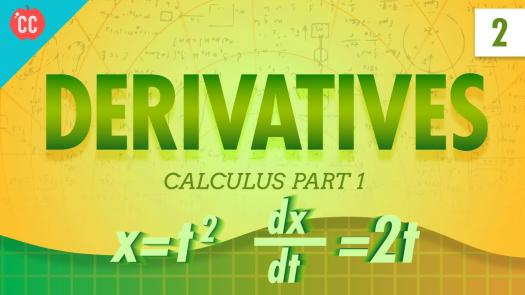# Do You Know Your Derivative Rules?

10 Questions | Total Attempts: 103SettingsDerivative rules count a number of rules that govern all the sets of derivatives in mathematics, like, for instance, derivatives of exponential and logarithmic function, derivatives of trigonometric functions, or again, derivatives of hyperbolic functions. If you believe you've always been a math genius, then have fun taking our quiz.

• 1.
What does the equation (d/dx) .(c^ax)= c^ax (lnc .a), c>0 mean?
• A.

That the equation above is true for all c, but the derivative for c=0 yields a complex number

• B.

That the equation above is true for all c, but the derivative for c>0 yields a complex number

• C.

That the equation above is true for all c, but the derivative for c

• D.

That the equation above is true for all c, but the derivative for c yields a complex number.

• 2.
What is the ideal number sentence describing logarithmic derivative?
• A.

Lnf= f/f

• B.

(lnf)'=f'/f

• C.

Lnf'=f/f'

• D.

Lnf'=f/1

• 3.
What are the 2 arguments that define an inverse tangent?
• A.

F'(x,y)

• B.

(y,x)

• C.

Arctan (y,x).

• D.

F(x)'

• 4.
What is the number sentence for the digamma function?
• A.

ψ0(x)

• B.

0(x)

• C.

ψ(x)

• D.

ψ0(x) +1

• 5.
What is the set of rules that can help compute the derivative function?
• A.

Fa di Bruno's theorem and the general Leibniz rule.

• B.

Faa di Bruno's formula and the general Leibniz rule.

• C.

Faa di Bruno's theorem and the general Leibniz theorem.

• D.

The Bruno's theorem and the Leibnis rule.

• 6.
What are the central objects of study in a complex analysis?
• A.

The polymorphic function

• B.

The holomorphic function

• C.

The morphological rule

• D.

The isomorphic rule

• 7.
What is the Schwarzian derivative?
• A.

It is one that describes how a function is approximated by a fractional-linear map.

• B.

It is one that describes how a complex function is approximated by a fractional-linear map.

• C.

It is one that describes how a simple function is approximated by a fractional-linear map.

• D.

It is one that describes how a compounded function is approximated by a fractional-linear map.

• 8.
Define the Wirtinger derivative? (pick the right answer.)
• A.

It's a set of differential operators permitting the construction of a differential calculus for complex functions.

• B.

It is one that completes a complex function is approximated by a fractional-linear map.

• C.

It is one that describes how a simple function is approximated by a fractional-linear map.

• D.

It is one that describes how a ordinary function is approximated by a fractional-linear map.

• 9.
What is the role of the functional derivative?
• A.

It is one with respect to a function of a functional on a space of formulas.

• B.

It is one with respect to a function of a functional on a space of functions.

• C.

It is one with respect to a function of a functional on a space of rules.

• D.

It is one with respect to a function of a functional on a space of domains.

• 10.
Explain the Frechet derivative?
• A.

It allows the extension of the directions derivatives to a general Banach space.

• B.

It allows the restrictions of the directions derivatives to a general Banach space.

• C.

It allows the construction of the directions derivatives to a general Banach space.

• D.

It shrinks the extension of the directions derivatives to a general Banach space.

Related TopicsBack to top